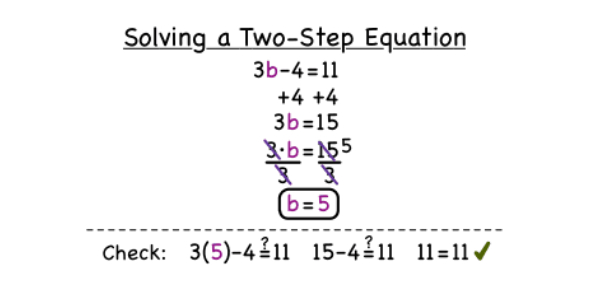# Solving Two Step Equations Quiz! Trivia

10 Questions | Attempts: 250
ShareSettings.

• 1.
4x + 12(x - 3) = 28
• A.

4x

• B.

-4

• C.

4

• D.

2

• 2.
X/2 + 13 = 20
• A.

27

• B.

66

• C.

14

• D.

-27

• 3.
-4x - 2 = 4
• A.

-1.5

• B.

1.5

• C.

15

• D.

32

• 4.
X/5 + 10 = 4/5
• A.

10

• B.

-46

• C.

5

• 5.
8 = -2/3(2x - 6)
• A.

6

• B.

3

• C.

-3

• D.

12

• 6.
Hemeron earns \$9 per hour at his after-school job.  On major holidays, such as Thanksgiving, he earns twice as much per hour.  He earned a total of \$405 for the week including Thanksgiving.  How many hours would Hemeron have to work on Thanksgiving if he worked 35 hours during the rest of the week?
• 7.
11x - 3x = 12 - 20
• 8.
5x - 14 = 21
• 9.
In the equation 7 - 2x = 15, the value of x is -4
• A.

True

• B.

False

• 10.
In the equation 4(x - 3) = 24, the value of x is -3
• A.

True

• B.

False

## Related TopicsBack to top
×

Wait!
Here's an interesting quiz for you.